Validate operation
Quantemplate allows validation rules and actions to be configured via a validation operation within a pipeline Transform stage. On running the pipeline the results are summarised in a Validation Report.

Validation rules are logical queries configured using Quantemplate’s Equation Editor. This allows flexible queries to be devised, such as checking that the values in a column do not exceed the values in another column, or that the length of a text string is within specified parameters.

If the result of the rule is ‘true’ (e.g. a text string is within a specified length) the validation will pass. If the result is ‘false’ the rule can be set to return a failure or a warning in the validation report.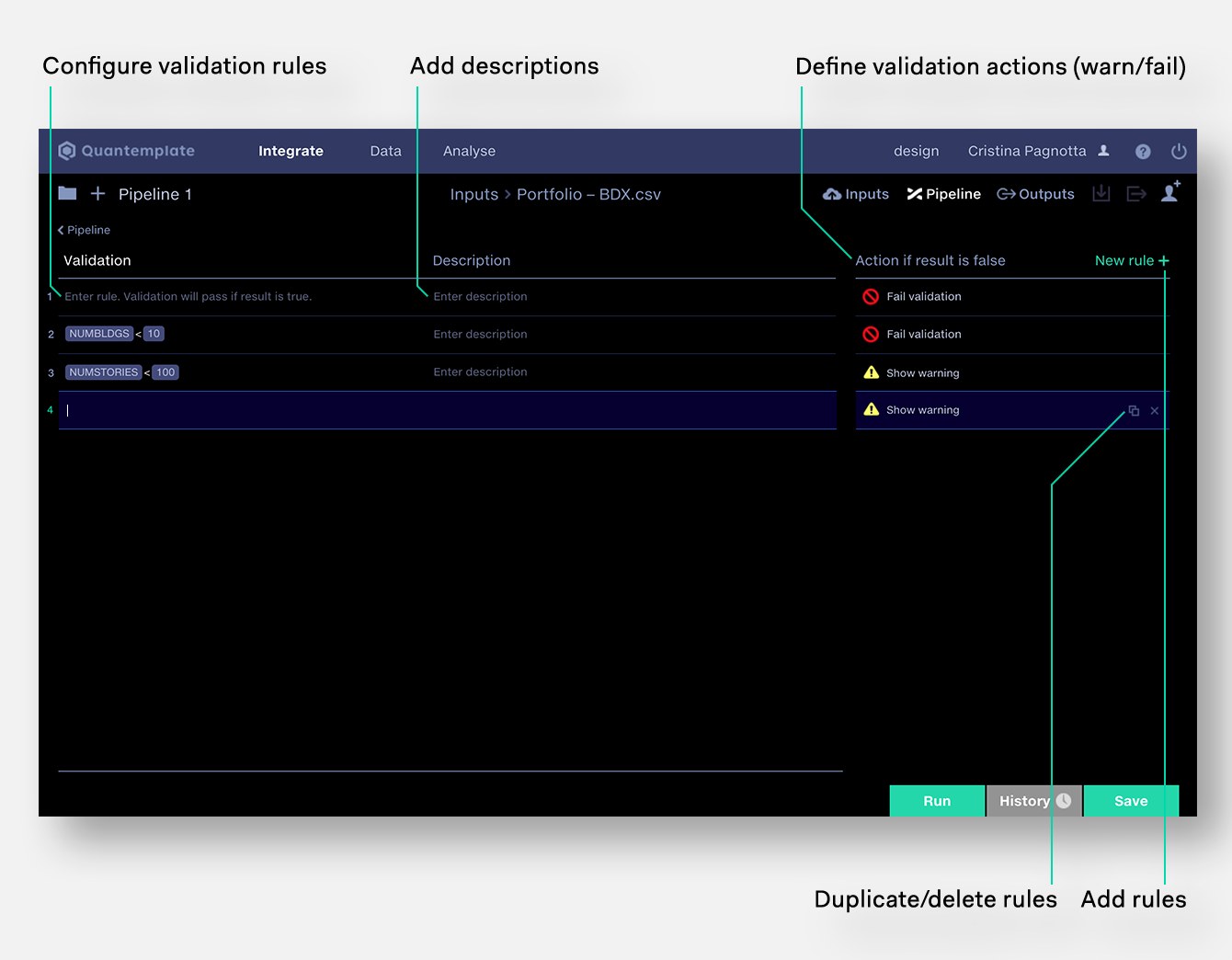1.
Open a transform stage and ensure inputs are configured.

2.
In the operations section click
+
to open the operations palette.

3.
Select
Validation

4.
Click on the operation to edit it.
Configuring validation rules
To configure a validation rule:

1.
Click on a row in the validation rules

2.
Select a column or function (fx) from the suggested list

3.
Add syntax, values or other columns to the equation

4.
Add a short text description to the rule, so it’s easy to see what the purpose of the rule is when viewing the Validation Report.

Rules can be duplicated, deleted or re-ordered.
Setting validation actions
If the result of a validation rule is ‘true’ the validation will pass. If the result is ‘false’ the rule can trigger a failure, a warning, or take no action.
Fail validation
Intended for validation results with erroneous values which adversely affect data quality.
Show warning
Intended for validation results which may be cause for concern possibly requiring further investigation.
No action
To disable a validation rule without deleting it, set ‘No action’. This can be useful for incrementally debugging a dataset
Example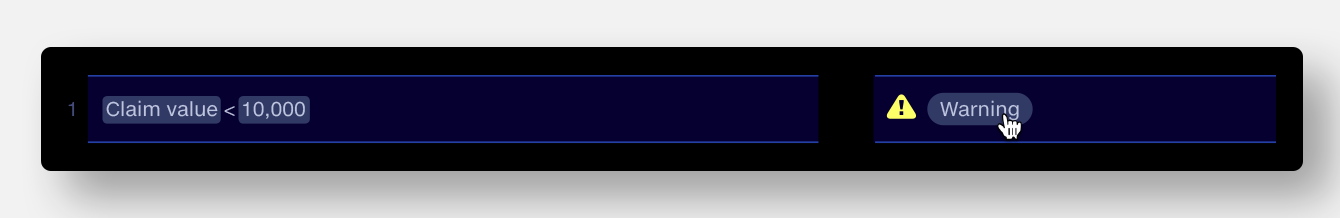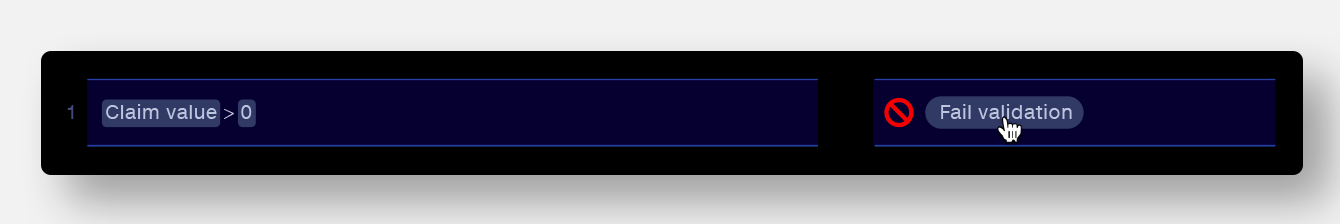In the ‘Claim value’ column, values less than 10,000 but greater than 0 will pass validation.

Values greater than or equal to 10,000 will show a warning.

Values less than 0 will fail validation.

Reordering validation rules
To reorder validation rules, hover over a drag handle at the right end of the validation rules row. Drag and drop the rule to its new position in the table. The new position will be reflected in the validation report.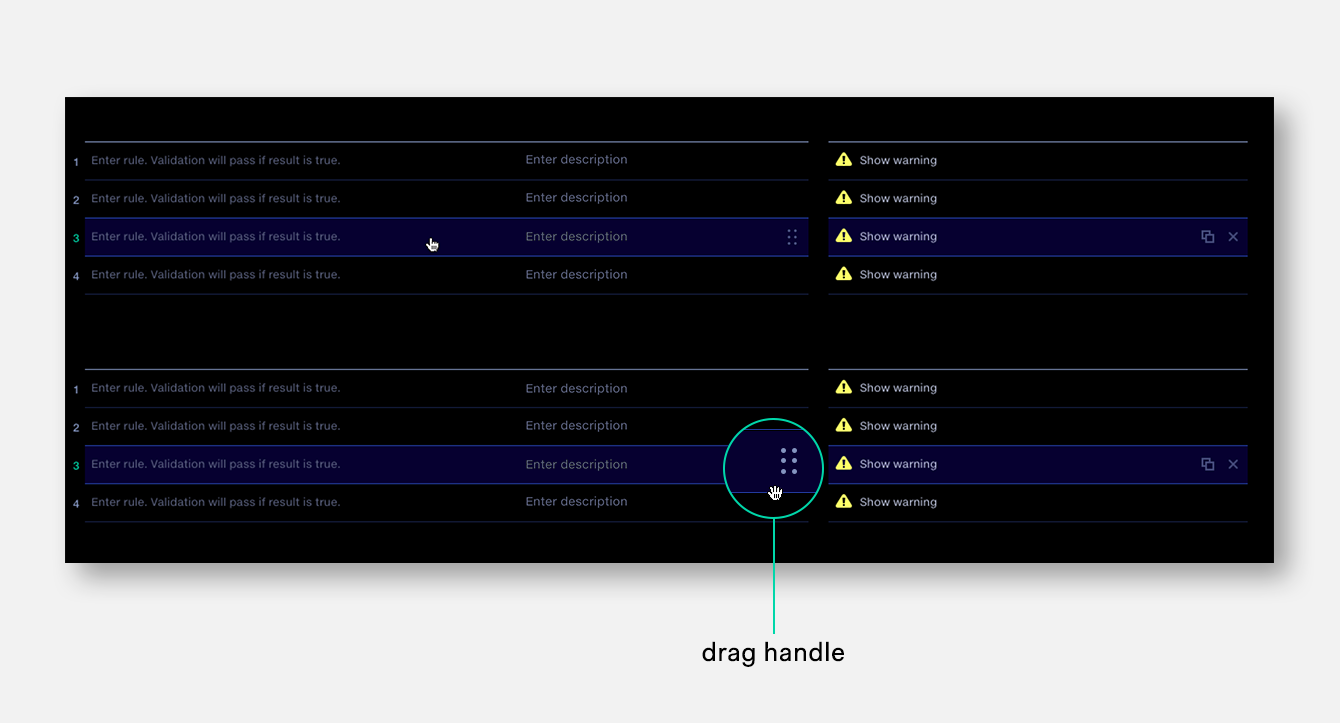Copying validation rules
Validation rules can be copied and pasted all at once to another validation operation. This is useful for duplicating a set of validation rules across multiple pipelines.
To copy rules: open a validation operation and with no rules selected click ctrl+c (Win) or cmd+c (Mac).
To paste rules: open a validation operation and click ctrl+v (Win) or cmd+v (Mac). This will add the copied rules below any existing rules.
To duplicate a validation operation: copy the rules from one operation, create a new validation operation and paste them in.
Note that the validation operation will need the same input column names for the rules to work.
Renaming the validation operation
It is sometimes useful to rename a validation operation. You may wish to do this to provide a more precise description of the nature of the validations in the operation, or to distinguish between multiple validation operations in a single stage.
To rename the validation operation:

1.
Enter the validation operation.

2.
Click on the ‘Validation’ heading top left.

3.
Type the new name.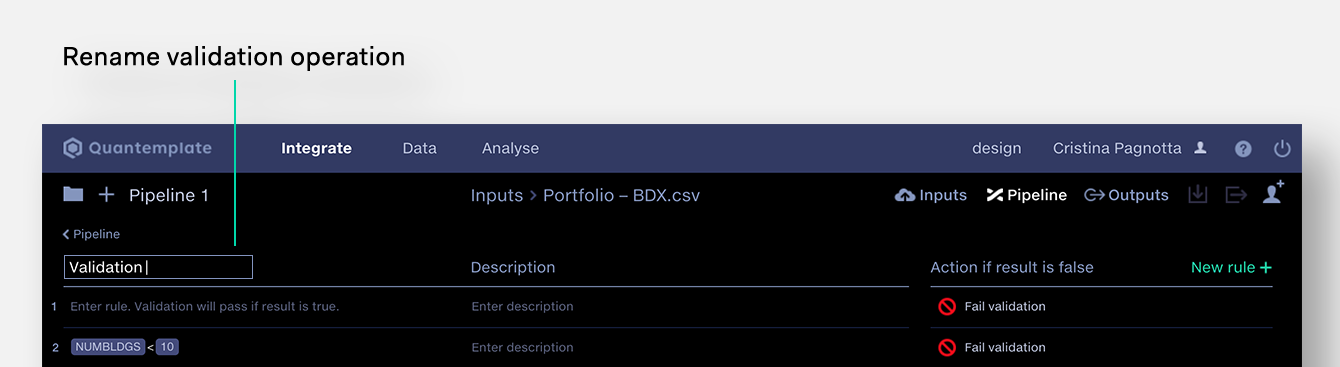Functions index
The Equation Editor in the Calculate and Validation operations supports a range of Excel-like functions for general purpose data cleansing and set of functions specifically for cleansing dates. To define columns, values and operators in functions, use the syntax described in the Operators and syntax section.
General Functions
ABS
Returns the absolute value of a number.
``ABS(<column>)``
Example
 VALUE ABS('VALUE') 1 1 -1 1
CONCATENATE
Joins multiple values together to create a single text value.
``CONCATENATE(<column name or value> … )``
Example
 ID CONCATENATE("X", 'ID') 123 X123 1234 X1234
FIND
Finds a text value and returns position of its first character.
``FIND(<search within>, <search for>)``
Note:
• The first character in the searched text is counted as position 1.
• If no match is found, the value 0 will be returned.
• Searching for a blank value will return 0.
• The match is case-sensitive. To perform a case-insensitive match combine with LOWER, UPPER or PROPER functions:
``FIND(UPPER(<search within>), UPPER(<search for>))``
Examples
Find the position of the character ‘d’ within the ID column.
 ID FIND('ID’, “d”) abcd 4 defg 1 efgh 0
Extract the first word of a text value, for example extracting a first name from a full name. Note that in this example, if the name does not contain a space, a blank will be returned.
 NAME LEFT('NAME', FIND('NAME', " ") - 1) Ronald Davies Ronald Jane Alice Smith Jane F. Scott Fitzgerald F. Madonna
Check whether a column contains a given text value, for example check whether a post code contains SE4.
 Postcode IF(FIND('Postcode', "SE4") > 0, "Yes", "No") SE4 5BX Yes SE13 4WZ No SE47RS Yes
Remove the first word, for example remove a building number from a street address. This expression looks for the position of the first space and returns everything after that space, returning ‘Unknown’ if no space is found.
 Street IF(FIND('Street', " ") = 0, "Unknown", MID('Street', FIND('Street', " ") + 1, LENGTH('Street'))) 23 Acacia Avenue Acacia Avenue 361–364 Park Road Park Road London Unknown
IF
Checks whether a condition is met and returns one value if true, and another value if false.
``IF(<logical query>, <value if true>, <value if false>)``
Examples of logical queries:
``````'Line_of_Business' = "Property"
'Region' = "Americas"
'Claim' > 1000000``````
IF statement example
If the Line of Business is Property, then multiply premium by 1.2. If it is not Property leave the Premium value unchanged.
``IF('Line_of_Business' = "Property", 'Premium' * 1.2, 'Premium')``
Using and/or in IF statements
And statement
``````IF('Column 1' > 'Column 2' and 'Column 3' < 'Column 4',
value_if_true, value_if_false)``````
Or statement
``````IF('Column 1' > 'Column 2' or 'Column 3' < 'Column 4',
value_if_true, value_if_false)``````
Combined statement
``````IF(('Column 1' > 'Column 2' or 'Column 1' < 'Column 5')
and ('Column 3' < 'Column 4'), value_if_true, value_if_false)``````
IFERROR
Returns a value if a calculation generates an error.
``IFERROR(<calculation>, <value>)``
Example
If the calculation ‘claim_amount / claim_count’ generates an error, return the value 0.
``IFERROR('claim_amount' / 'claim_count', 0)``
ISNUMBER
Returns TRUE if value is a number, and FALSE if not (for use with IF statements).
``ISNUMBER(<column>)``
Example
 value IF(ISNUMBER('value'), "Number", "Not a number") 1 Number "1" Number 1.2 Number "" Not a number "text" Not a number
ISLIKE
Returns TRUE if value matches a regular expression, and FALSE if not (for use with IF statements)
``ISLIKE(<column>, "regular expression")``
Example
 ID IF(ISLIKE('ID', "4\$"), "Ends with 4", "Doesn't end with 4") 123 Doesn’t end with 4 1234 Ends with 4
LEFT
Returns the first characters from a value, given the number of characters
``LEFT(<column>, <no. of characters>)``
Example
 Address LEFT('SIC Code', 4) 0800 Forestry 0800 2080 Beverages 2080
LENGTH
Returns the length of each column value.
``LENGTH(<column>)``
Example
 ID LENGTH('ID') 123 3 1234 4
LOWER
Converts text to lowercase.
``LOWER(<column name or text value> … )``
MAX
Returns the maximum value across multiple columns or constant values.
Each parameter can be a numeric value or a column name. If the parameter is a column name then the function will get the value in that column. All the values are compared and the largest value is returned.
``MAX(<column name or numeric value>, … )``
Example
 VALUE MAX('VALUE',0) 1 1 -1 0
MID
Returns characters from a value, given starting position (where first character is 1) and number of characters.
``MID(<column>, <start position>, <no. of characters>)``
Example
 ID MID('ID', 4, 2) 12345678 45 abcdefghijkl de
MIN
Returns the minimum value across multiple columns or constant values.
Each parameter can be a numeric value or a column name. If the parameter is a column name then the function will get the value in that column. All the values are compared and the smallest value is returned.
``MIN(<column name or numeric value>, … )``
Example
 VALUE MIN('VALUE',0) 1 0 -1 -1
PROPER
Converts the first letter of each word to uppercase and the other letters to lowercase.
``PROPER(<column name or text value> … )``
Example
 VALUE PROPER('VALUE') SAN FRANCISCO San Francisco sao paulo Sao Paulo SAN DIEGO, CA San Diego, Ca
Returns the last characters from a value, given the number of characters
``RIGHT(<column>, <no. of characters>)``
Example
 Address RIGHT('Address', 8) London, EC1Y 1AA EC1Y 1AA Penzance, TR19 7AA TR19 7AA
ROUND
Rounds a number to a given number of digits.
``ROUND(<column name or numeric value>, <no. of digits>)``
Numbers 1–4 are rounded down and 5–9 are rounded up. Numbers are rounded to a specified level of precision. They can be rounded to the right or left of the decimal point.
If num_digits > 0, number is rounded to the specified number of decimal places to the right of the decimal point.

If num_digits < 0, number is rounded to the left of the decimal point (i.e. to the nearest 10, 100, 1000, etc.).

If num_digits = 0, number is rounded to the nearest integer.

Examples
 Formula Description Result ROUND(3.14, 1) Rounds 3.14 to one decimal place 3.1 ROUND(12.34, -1) Rounds 12.34 to one decimal place to the left of the decimal point 10 ROUND(876.5, -3) Rounds 876.5 to the nearest 1000 1000
SUBSTITUTE
Finds a text value and replaces it with another text value.
``SUBSTITUTE(<search within>, <search for>, <replace with>)``
Note:
• All instances of the searched for text in the searched within text will be replaced.
• If no searched for text is found, the searched within text is not changed.
• If the searched within text is blank, the result will be blank.
• If the searched for text is blank, the result will be the searched within text, unchanged.
• If the replace with text is blank then the searched for text will be removed.
• The match is case-sensitive.
Example
Remove dashes from an ID value.
 ID SUBSTITUTE('ID', "-", "") QUAN-1234-785 QUAN1234785 QUAN7436528 QUAN7436528
SUBSTRING
Returns characters from a value, given starting position (where first character is 0) and number of characters.
``SUBSTRING(<column>, <start position>, <no. of characters>)``
Example
 ID SUBSTRING('ID', 4, 2) 12345678 56 abcdefghijkl ef
TRIM
Removes extra spaces from text: leading and trailing whitespace and repeated spaces between words.
``TRIM(<column name or text value> … )``
UPPER
Converts text to uppercase.
``UPPER(<column name or text value> … )``
Date Functions
Introducing the new date functions
Date functions supersede the legacy script operations Add term to date and Duration. These operations will soon be deprecated so they can no longer be added to a pipeline. They will continue to work in existing pipelines. Updating existing pipelines to remove legacy date operations is advised.
Date functions operate on cleansed date values which are in Basic ISO format
yyyymmdd
.
• Blank date values are left blank
• Date values that are not in Basic ISO format will cause the pipeline run to fail.
DATECALC
Returns a new basic-ISO formatted date by adding or removing a term (constant value or column reference) from a basic-ISO date.
``DATECALC(<iso date>, <term (numeric value)>, <unit>)``
• The term is rounded to the lowest whole number (negative values are rounded up towards zero).
• Adding or subtracting by month/year to a day that is invalid (e.g. to 31st April) will truncate to the last valid day of that month
• <unit> is one of "d"/"day"/"days"; "m"/"month"/"months"; or "y"/"year"/"years"
Example
 Premium DATECALC('Premium', -1, "year") DATECALC('Premium', 6, "months") 20120629 20110629 20121229 20200229 20190228 20200829 "" "" "" 2012-06-29 (pipeline error) (pipeline error)
DURATION
Returns the number of whole elapsed units between two basic-ISO dates.
``DURATION(<start>, <end>, <unit>)``
• The result is rounded to the lowest whole number (negative values are rounded up towards zero).
• If the end is before the start, a negative number will be returned.
• <unit> is one of "d"/"day"/"days"; "m"/"month"/"months"; or "y"/"year"/"years"
Example
 Start End DURATION('Start', 'End', "days") 20120629 20120630 1 20120630 20120629 -1 "" "" "" 2012-06-29 2012-06-30 (pipeline error)
ENDOFQUARTER
Moves a date to the last day in a quarter.
``ENDOFQUARTER(<iso date>)``
ENDOFMONTH
Moves a date to the last day in a month.
``ENDOFMONTH(<iso date>)``
ENDOFYEAR
Moves a date to the last day in a year.
``ENDOFYEAR(<iso date>)``
STARTOFQUARTER
Moves a date to the first day in a quarter.
``STARTOFQUARTER(<iso date>)``
STARTOFMONTH
Moves a date to the first day in a month.
``STARTOFMONTH(<iso date>)``
STARTOFYEAR
Moves a date to the first day in a year.
``STARTOFYEAR(<iso date>)``
Operators and syntax
Valid operators in the equation editor:
+
plus

-
minus

/
divide

*
multiply

^
exponent

=
equals

!=
not equals

>
greater than

<
less than

>=
greater than or equal to

<=
less than or equal to
Standard syntax must be followed for the equation editor to function. Quantemplate automatically inserts correct syntax where possible.
‘ ’

“ ”
IF(...)
,